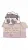Crownnote is where music fans share their favorite songs.

Chart

# END OF YEAR 2022

ALBUM CHART

1.Dawn FM - The Weeknd
2.CRASH - Charli XCX
3.Happier Than Ever - Billie Eilish
4.Red (Taylor's Version) - Taylor Swift
5.Midnights - Taylor Swift
6.WHEN WE ALL FALL ASLEEP, WHERE DO WE GO? - Billie Eilish
7.Harry's House - Harry Styles
8.Mercury (Acts 1 & 2) - Imagine Dragons
9.Mr Morale & The Big Steppers - Kendrick Lamar
10.folklore - Taylor Swift
11.Blurryface - Twenty One Pilots
12.The Walls Are Way Too Thin - Holly Humberstone
13.MONTERO - Lil Nas X
14.If I Can't Have Love, I Want Power - Halsey
15.1989 - Taylor Swift
16.You Signed Up For This - Maisie Peters
17.evermore - Taylor Swift
18.Speak Now - Taylor Swift
19.SOUR - Olivia Rodrigo
20.Trench - Twenty One Pilots

Honourable mentions:

• Blue Weekend - Wolf Alice
• Optimist - FINNEAS
• Oracular Spectacular - MGMT
• SOS - SZA
• This Is What I Mean - Stormzy
1. Number
1
2. Number
2
3. Number
3
4. Number
4
5. Number
5
6. Number
6
7. Number
7
8. Number
8
9. Number
9
10. Number
10
11. Number
11
12. Number
12
13. Number
13
14. Number
14
15. Number
15
16. Number
16
17. Number
17
18. Number
18
19. Number
19
20. Number
20
21. Number
21
22. Number
22
23. Number
23
24. Number
24
25. Number
25
26. Number
26
27. Number
27
28. Number
28
29. Number
29
30. Number
30
31. Number
31
32. Number
32
33. Number
33
34. Number
34
35. Number
35
36. Number
36
37. Number
37
38. Number
38
39. Number
39
40. Number
40
41. Number
41
42. Number
42
43. Number
43
44. Number
44
45. Number
45
46. Number
46
47. Number
47
48. Number
48
49. Number
49
50. Number
50
51. Number
51
52. Number
52
53. Number
53
54. Number
54
55. Number
55
56. Number
56
57. Number
57
58. Number
58
59. Number
59
60. Number
60
61. Number
61
62. Number
62
63. Number
63
64. Number
64
65. Number
65
66. Number
66
67. Number
67
68. Number
68
69. Number
69
70. Number
70
71. Number
71
72. Number
72
73. Number
73
74. Number
74
75. Number
75
76. Number
76
77. Number
77## The Very First Night (Taylor's Version) (From The Vault)

From the album: Red (Taylor's Version)

78. Number
78
79. Number
79
80. Number
80
81. Number
81
82. Number
82
83. Number
83
84. Number
84
85. Number
85
86. Number
86
87. Number
87
88. Number
88
89. Number
89
90. Number
90
91. Number
91
92. Number
92
93. Number
93
94. Number
94
95. Number
95
96. Number
96
97. Number
97
98. Number
98
99. Number
99
100. Number
100
101. Dropouts
1. Number
2 Dropout
Peak
2
Weeks
7
2. Number
3 Dropout
Peak
1 (for 1 weeks)
Weeks
4
3. Number
4 Dropout
Peak
3
Weeks
3
4. Number
5 Dropout
Peak
5
Weeks
4
5. Number
7 Dropout
Peak
7
Weeks
3
6. Number
8 Dropout
Peak
1 (for 1 weeks)
Weeks
8
7. Number
9 Dropout
Peak
5
Weeks
8
8. Number
10 Dropout
Peak
4
Weeks
7

A chart byethan i
Date
Songs
100
No points